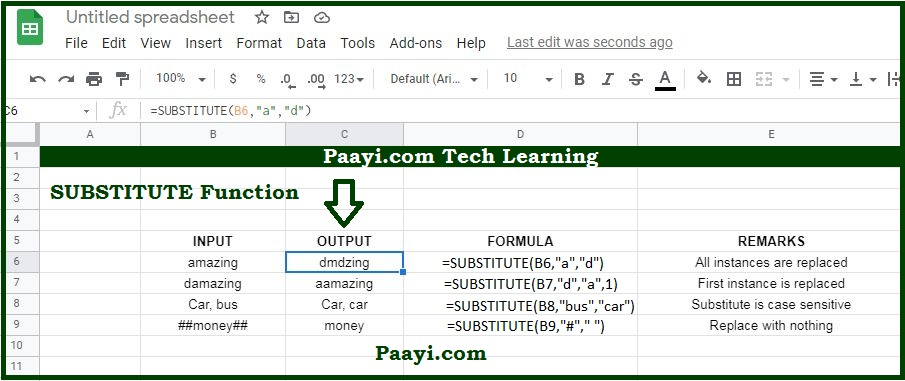# Learn How to Use Google Sheets SUBSTITUTE Function

Written by | 0 Comments | 860 Views

In this article, you will learn how to use a Google sheets SUBSTITUTE function and its prime function in Google sheets. You will also get to know the Google sheets SUBSTITUTE function return value and syntax with the help of some examples.

The Google sheets SUBSTITUTE function is used to replace text based on the content. That means with the help of the SUBSTITUTE function; you can able to replace a text in the given text string by matching.

Let us understand this by the example given here:

= SUBSTITUTE("937-328-8832", "-", " ") It will return the value 9373288432, and dash are removed. The SUBSTITUTE function does support wild cards, and it is case-sensitive.

### SUBSTITUTE Function Return Value

The return value will be the processed text.

### SUBSTITUTE Function Syntax

= SUBSTITUTE(text, old-text, new-text, [instance])

Where the arguments:

• text: It is the text you want to change.
• old-text: This is the text you want to replace.
• new-text: The is the new text you want to replace with.
• instance: It is the instance to replace; if not provided, all instances will be replaced (Optional).

## How to Use Google Sheets SUBSTITUTE Function?The Google sheet SUBSTITUTE function can replace text by matching. The SUBSTITUTE function can be used when you want to replace the text based on its content and not position. You have the option to specify the instance of the found text to replace, that is, first instance, second instance, etc. Let us understand the SUBSTITUTE function by the examples provided below:

Example 1: = SUSBSTITUTE(B6, "t", "b") - This will replace all be t's with b's.

Example 2: = SUSBSTITUTE(B7, "t", "b", 1) - This will replace first "t" with "b."